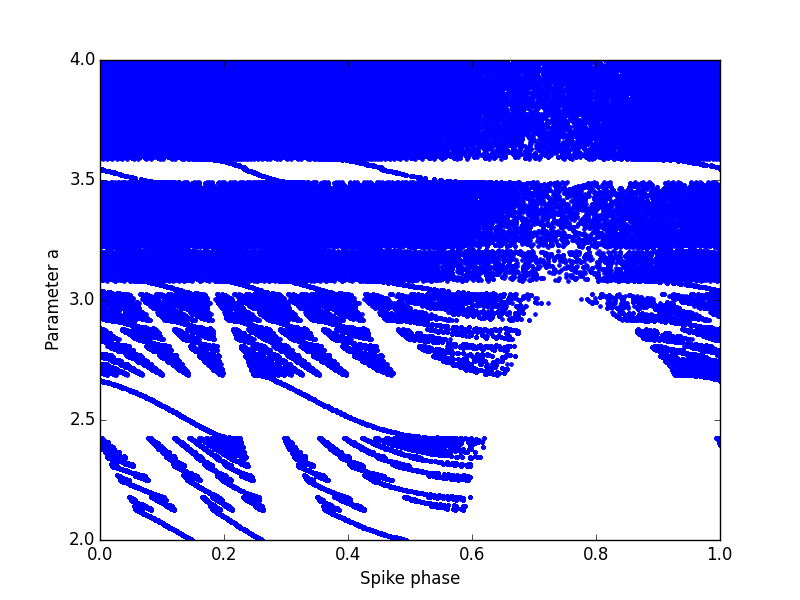# Example: Brette_2004¶

Note

You can launch an interactive, editable version of this example without installing any local files using the Binder service (although note that at some times this may be slow or fail to open):## Phase locking in leaky integrate-and-fire model¶

Fig. 2A from: Brette R (2004). Dynamics of one-dimensional spiking neuron models. J Math Biol 48(1): 38-56.

This shows the phase-locking structure of a LIF driven by a sinusoidal current. When the current crosses the threshold (a<3), the model almost always phase locks (in a measure-theoretical sense).

from brian2 import *

# defaultclock.dt = 0.01*ms  # for a more precise picture
N = 2000
tau = 100*ms
freq = 1/tau

eqs = '''
dv/dt = (-v + a + 2*sin(2*pi*t/tau))/tau : 1
a : 1
'''

neurons = NeuronGroup(N, eqs, threshold='v>1', reset='v=0', method='euler')
neurons.a = linspace(2, 4, N)

run(5*second, report='text')  # discard the first spikes (wait for convergence)
S = SpikeMonitor(neurons)
run(5*second, report='text')

i, t = S.it
plot((t % tau)/tau, neurons.a[i], '.')
xlabel('Spike phase')
ylabel('Parameter a')
show()